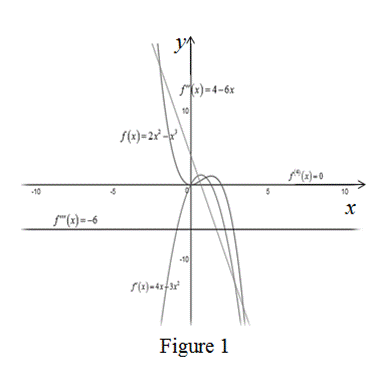# The first, second, third and fourth derivatives of the function f ( x ) = 2 x 2 − x 3 .### Single Variable Calculus: Concepts...

4th Edition
James Stewart
Publisher: Cengage Learning
ISBN: 9781337687805### Single Variable Calculus: Concepts...

4th Edition
James Stewart
Publisher: Cengage Learning
ISBN: 9781337687805

#### Solutions

Chapter 2.7, Problem 47E
To determine

## To find: The first, second, third and fourth derivatives of the function f(x)=2x2−x3.

Expert Solution

The first, second, third and fourth derivatives of the function is f(x)=4x3x2, f(x)=46x, f(x)=46x and f(4)(x)=6.

### Explanation of Solution

Given:

The function is, f(x)=2x2x3.

Result Used:

The derivative of a function f is denoted by f(x) is,

f(x)=limh0f(x+h)f(x)h (1)

Calculation:

The first derivative of the function is computed as follows,

f(x)=limh0f(x+h)f(x)h=limh02(x+h)2(x+h)3{2x2x3}h=limh02x2+2h2+4xhx3h33x2h3xh22x2+x3h=limh02h2+4xhh33x2h3xh2h

Simplified further to obtain the derivative of the function,

f(x)=limh0(2h+4xh23x23xh)=2(0)+4x(0)23x23x(0)=4x3x2

Therefore, the derivative of the function f(x) is, f(x)=4x3x2.

The second derivative of the function is computed as follows,

f(x)=(f(x))=limh0f(x+h)f(x)h=limh04(x+h)3(x+h)2{4x3x2}h=limh04x+4h3x23h26xh4x+3x2h

Simplify the expression and obtain the derivatives,

f(x)=limh04h3h26xhh=limh0(43h6x)=43(0)6x=46x

Therefore, the second derivative of the function f(x) is, f(x)=46x.

The third derivative of the function is computed as follows,

f(x)=(f(x))=limh0f(x+h)f(x)h=limh046(x+h){46x}h=limh046x6h4+6xh

Simplify the terms and obtain derivatives,

f(x)=limh06hh=6limh0hh=6limh01=6

Therefore, the third derivative of the function f(x) is, f(x)=6.

The fourth derivative of the function is computed as follows,

f(4)(x)=(f(x))=limh0f(x+h)f(x)h=limh06{6}h=limh0(0)

=0

Thus, the fourth derivative of the function f(x) is f(4)(x) =0.

To sketch: The graph of the functions f(x),f(x),f(x) and f(x) and explain the graphs consistent with the geometric interpretations of these derivatives.

The graphs are consistent with the geometric interpretations of these derivatives.

Graph:

Use the online graphing calculator to draw the functions f(x),f(x),f(x),f(x) and f(4)(x) are shown below in Figure 1.Geometrical Interpretation:

Take several points on the domain and estimate slope of the tangent to the function f(x) which is same as the point of f(x) at that point.

Thus, the derivative f(x) is reasonable.

The f(x) represents a quadratic function and f(x) represents a linear function.

Take several points on the domain and estimate slope of the tangent to the function f(x) which is same as the point of f(x) at that point.

Therefore, the function f(x) is reasonable.

The f(x) represents a linear function and f(x) represents a constant function.

The straight line has same slope at all points. That is, f(x) is constant value.

From the Figure 1, the estimated slope of the function f(x) is 6 which is same as the derivative of f(x). That is, f(x)=6.

Therefore, the function f(x) is reasonable.

### Have a homework question?

Subscribe to bartleby learn! Ask subject matter experts 30 homework questions each month. Plus, you’ll have access to millions of step-by-step textbook answers!